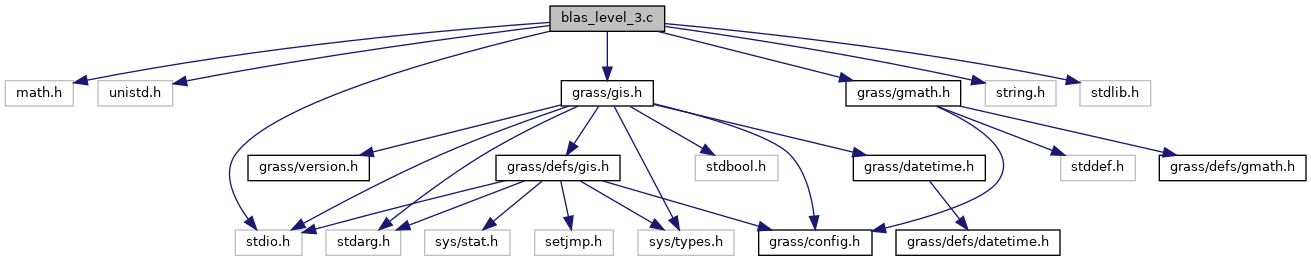GRASS GIS 7 Programmer's Manual  7.9.dev(2021)-e5379bbd7
blas_level_3.c File Reference
#include <math.h>
#include <unistd.h>
#include <stdio.h>
#include <string.h>
#include <stdlib.h>
#include <grass/gis.h>
#include <grass/gmath.h>
Include dependency graph for blas_level_3.c:Go to the source code of this file.

## Functions

void G_math_d_aA_B (double **A, double **B, double a, double **C, int rows, int cols)
Add two matrices and scale matrix A with the scalar a. More...

void G_math_f_aA_B (float **A, float **B, float a, float **C, int rows, int cols)
Add two matrices and scale matrix A with the scalar a. More...

void G_math_d_AB (double **A, double **B, double **C, int rows_A, int cols_A, int cols_B)
Matrix multiplication. More...

void G_math_f_AB (float **A, float **B, float **C, int rows_A, int cols_A, int cols_B)
Matrix multiplication. More...

## ◆ G_math_d_aA_B()

 void G_math_d_aA_B ( double ** A, double ** B, double a, double ** C, int rows, int cols )

Add two matrices and scale matrix A with the scalar a.In case B == NULL, matrix A will be scaled by scalar a.
In case a == 1.0, a simple matrix addition is performed.
In case a == -1.0 matrix A is subtracted from matrix B.
The result is written into matrix C.

This function is multi-threaded with OpenMP and can be called within a parallel OpenMP region.

Parameters
 A (double **) B (double **) if NULL, matrix A is scaled by scalar a only a (double) C (double **) rows (int) cols (int)
Returns
(void)

Definition at line 50 of file blas_level_3.c.

## ◆ G_math_d_AB()

 void G_math_d_AB ( double ** A, double ** B, double ** C, int rows_A, int cols_A, int cols_B )

Matrix multiplication.The result is written into matrix C.

A must be of size rows_A * cols_A B must be of size rows_B * cols_B with rows_B == cols_A C must be of size rows_A * cols_B

This function is multi-threaded with OpenMP and can be called within a parallel OpenMP region.

Parameters
 A (double **) B (double **) C (double **) rows_A (int) cols_A (int) cols_B (int)
Returns
(void)

Definition at line 174 of file blas_level_3.c.

## ◆ G_math_f_aA_B()

 void G_math_f_aA_B ( float ** A, float ** B, float a, float ** C, int rows, int cols )

Add two matrices and scale matrix A with the scalar a.In case B == NULL, matrix A will be scaled by scalar a.
In case a == 1.0, a simple matrix addition is performed.
In case a == -1.0 matrix A is subtracted from matrix B.
The result is written into matrix C.

This function is multi-threaded with OpenMP and can be called within a parallel OpenMP region.

Parameters
 A (float **) B (float **) if NULL, matrix A is scaled by scalar a only a (float) C (float **) rows (int) cols (int)
Returns
(void)

Definition at line 113 of file blas_level_3.c.

## ◆ G_math_f_AB()

 void G_math_f_AB ( float ** A, float ** B, float ** C, int rows_A, int cols_A, int cols_B )

Matrix multiplication.The result is written into matrix C.

A must be of size rows_A * cols_A B must be of size rows_B * cols_B with rows_B == cols_A C must be of size rows_A * cols_B

This function is multi-threaded with OpenMP and can be called within a parallel OpenMP region.

Parameters
 A (float **) B (float **) C (float **) rows_A (int) cols_A (int) cols_B (int)
Returns
(void)

Definition at line 215 of file blas_level_3.c.